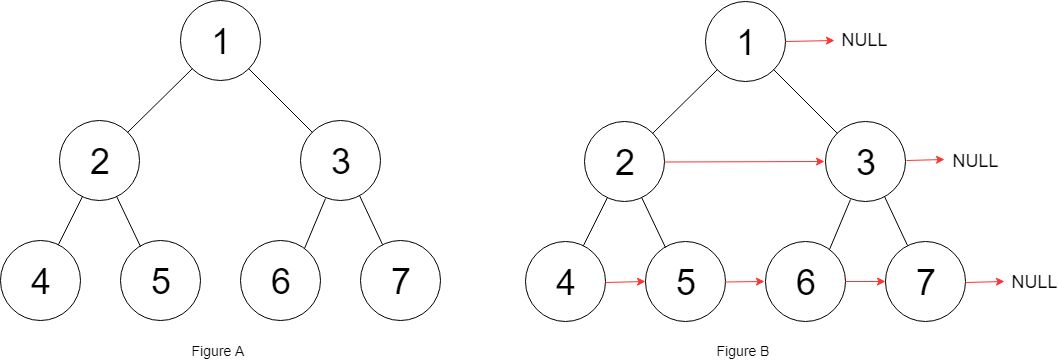# Introduction

Write a function to connect all the adjacent nodes at the same level in a binary tree.

``````Input Tree
A
/ \
B   C
/ \   \
D   E   F

Output Tree
A--->NULL
/ \
B-->C-->NULL
/ \   \
D-->E-->F-->NULL``````Example of setting the next node on the same level

# Approach

The suggested approach is to use the Level Order Traversal and set the next pointer.Example where nr represents the next pointer to be createdWe use queue and then set the next pointer for the elements
``````/*
// Definition for a Node.
public class Node {
public int val;
public Node left;
public Node right;
public Node next;

public Node() {}

public Node(int _val) {
val = _val;
}

public Node(int _val, Node _left, Node _right, Node _next) {
val = _val;
left = _left;
right = _right;
next = _next;
}
}
*/

public class Solution {
public Node Connect(Node root) {
if (root == null) {
return root;
}
Queue<Node> q = new Queue<Node>();
if (root.left != null) {
q.Enqueue (root.left);
}
if (root.right != null) {
q.Enqueue (root.right);
}

Node curr = null;
// Level order traversal
while (q.Count > 0) {
var qCount = q.Count;
while (qCount-- > 0) {
var prev = curr;
curr = q.Dequeue();
// Connect prev with curr
if (prev != null) {
prev.next = curr;
}

// Enqueue Left
if (curr.left != null) {
q.Enqueue(curr.left);
}
// Enqueue Right
if (curr.right != null) {
q.Enqueue(curr.right);
}
}
// Reset for the level
curr = null;
}
return root;
}
}``````